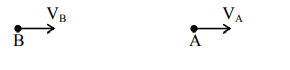Deepak Scored 45->99%ile with Bounce Back Crack Course. You can do it too!

# Car B overtakes another car A at a relative speed of

Question:

Car B overtakes another car A at a relative speed of $40 \mathrm{~ms}^{-1}$. How fast will the image of car B appear to move in the mirror of focal length $10 \mathrm{~cm}$ fitted in car $\mathrm{A}$, when the car $\mathrm{B}$ is $1.9 \mathrm{~m}$ away from the car A?

1. $4 \mathrm{~ms}^{-1}$

2. $0.2 \mathrm{~ms}^{-1}$

3. $40 \mathrm{~ms}^{-1}$

4. $0.1 \mathrm{~ms}^{-1}$

Correct Option: , 4

Solution:Mirror used is convex mirror (rear-view mirror)

$\therefore \mathrm{V}_{\mathrm{L} / \mathrm{m}}=-\mathrm{m}^{2} \mathrm{~V}_{\mathrm{O} / \mathrm{m}}$

Given,

$\mathrm{V}_{\mathrm{O} / \mathrm{m}}=40 \mathrm{~m} / \mathrm{s}$

$m=\frac{f}{f-u}=\frac{10}{10+190}=\frac{10}{200}$

$\therefore \mathrm{V}_{1 / \mathrm{m}}=-\frac{1}{400} \times 40=-0.1 \mathrm{~m} / \mathrm{s}$

$\therefore$ Car will appear to move with speed $0.1 \mathrm{~m} / \mathrm{s}$.

Hence option (4)